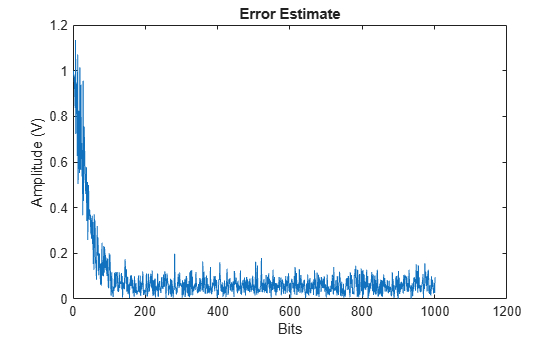# Equalize BSPK Signal

Equalize a BPSK signal using a linear equalizer with a least mean square (LMS) algorithm.

Generate random binary data and apply BPSK modulation.

```M = 2; data = randi([0 1],1000,1); modData = pskmod(data,M);```

Apply two-tap static fading to the modulated signal and add AWGN noise.

```rxSig = conv(modData,[0.02+0.5i 0.05]); rxSig = awgn(rxSig,30);```

Create a linear equalizer System object™ configured to use the LMS adaptive algorithm, 8 taps, 0.1 step size, and the 4th tap as the reference tap. Set the constellation to match the modulation of the transmitted signal.

```lineq = comm.LinearEqualizer( ... NumTaps=8, ... StepSize=0.1, ... Constellation=complex([-1 1]), ... ReferenceTap=4)```
```lineq = comm.LinearEqualizer with properties: Algorithm: 'LMS' NumTaps: 8 StepSize: 0.1000 Constellation: [-1.0000 + 0.0000i 1.0000 + 0.0000i] ReferenceTap: 4 InputDelay: 0 InputSamplesPerSymbol: 1 TrainingFlagInputPort: false AdaptAfterTraining: true InitialWeightsSource: 'Auto' WeightUpdatePeriod: 1 ```

Equalize the received signal, `rxSig`. Use the first 200 data bits as a training sequence. Display a constellation diagram showing the received signal before and after equalization.

```trSeq = modData(1:200); [eqSig,err] = lineq(rxSig,trSeq); constdiag = comm.ConstellationDiagram( ... NumInputPorts=2, ... ChannelNames={'Before equalization','After equalization'}, ... ReferenceConstellation=pskmod([0 M-1],M)); constdiag(rxSig(400:end),eqSig(400:end))```Plot the magnitude of the error estimate. As shown by the decrease and stabilizing of the error signal, the equalization converges in less than 200 bits.

```plot(abs(err)) title('Error Estimate') xlabel('Bits') ylabel('Amplitude (V)')```